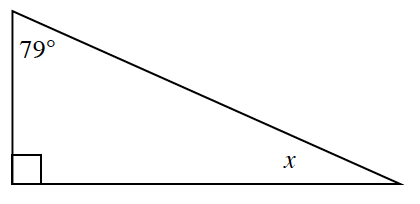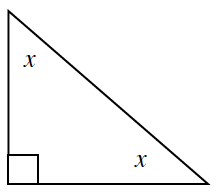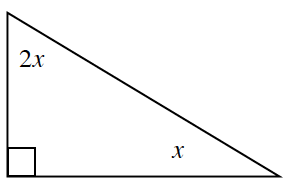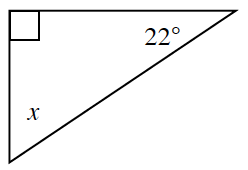### Home > CCG > Chapter 4 > Lesson 4.1.1 > Problem4-6

4-6.

Use what you know about the angles of a triangle to find the value of $x$ and the angles in each triangle below.

Use the Triangle Angle Sum Theorem, explained in the Math Notes box in Lesson 2.2.1.

1.$x+79º+90º=180º$

1.$x+x+90º=180º$

1.$x=30º$

1.$x=68º$